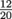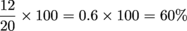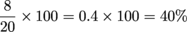## Percent Increase & Decrease

When an item goes on sale, or rises in price, it goes up or down by a certain percentage. This percentage is often referred to as a percent decrease or increase.

Flashing lights, blaring siren: warning, warning. This is different from finding what percent the new cost is of the old cost. In those problems, we divide the new price by the old price. In percent increase/decrease problems, we aren't comparing new and old prices; we're comparing the change of the new price to the old price. We write this as a fraction of the amount something changed over the amount it originally was. Then we need to change this fraction into a decimal, and then into a percent.

To find a percent increase or decrease, all we need to do is take the difference in amount and divide it by the original amount, which gives us a decimal. Then we multiply this by 100, which turns the decimal into a percent.### Sample Problem

If a game for our gaming system was originally \$20.00 and is now on sale for \$12.00, what percent is the new price of the old price? By what percent did the price decrease?

Both questions involve a fraction. The percent that the new price is of the old price is represented with the fraction. Now we divide 12 by 20, and then convert it into a percent by multiplying by 100.So the sale price is 60% of the original price, because \$12 is 60% of \$20.

To find the percent decrease, first we subtract \$12 from \$20 to see how much the price decreased by.

20 – 12 = 8

The sale price is 8 bucks less than the original price. Now we divide the amount it decreased, or \$8, by the original price of \$20. That gives us a decimal, which we then multiply by 100 to get a percent.The price decreased by 40%. In other words, the game was on sale for 40% off. That's a screamin' deal.